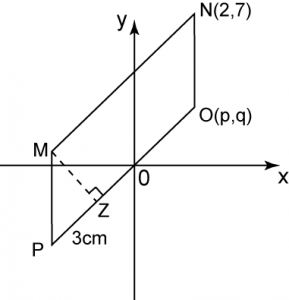# 5.7.3 The Straight Line, SPM Paper 2

Question 7:The diagram above shows a parallelogram on a Cartesian plane. MP and NO are parallel to the y-axis. Given that the distance of MZ is 4 units. Find
(a) the value of p and q.
(b) the equation of the straight line MN.

Solution:

Question 8:The diagram above shows that two straight line intersect at point (0 , -2). Find
(a) the value of b
(b) the x-intercept of the straight line XY if the gradient of XY is equal to 2.
(c) the equation of XY.

Solution:
(a)
Value of b
= 2 units + 3 units
= 5 units
= –5

Question 9:The diagram above shows that the straight line PMQ intersects with PNR at N. Given that OQ = OR and M is the midpoint of PQ. Find
(a) the coordinate of P
(b) the value of m and n.

Solution: